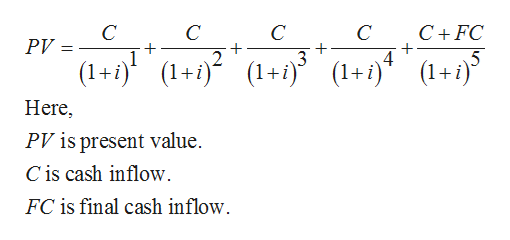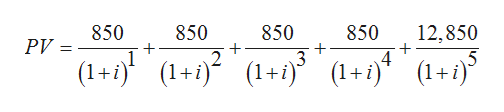Your father paid \$10,000 (cf occurs at t=0) for an investment that promises to pay \$850 at the end of each of the next 5 years, then an additional lump sum payment of \$12,000 at the end of the 5th year. What is the expected rate of return on this investment?

Question

Your father paid \$10,000 (cf occurs at t=0) for an investment that promises to pay \$850 at the end of each of the next 5 years, then an additional lump sum payment of \$12,000 at the end of the 5th year. What is the expected rate of return on this investment?

Step 1

Expected rate of return can be described as the total profit or loss on a particular investment over a set period of time. Expected rate of return is generally stated as a percentage in relation to primary cost of investment.

It is given that,

Initial cash outflow is \$10,000.

Annual cash inflow is \$850.

Cash inflow at the end of year 5 is \$12,000.

Step 2

The formula to calculate present value will be helpful to calculate the expected interest rate.

The below expression can be used to calculate expected interest rate:help_outlineImage TranscriptioncloseC C C C FC PV 3 5 (1+) (1+(+ (1+i)4(1+ij Here, PV is present value C is cash inflow FC is final cash inflow. fullscreen
Step 3

Substitute \$850 for C and \$12,000 for ...help_outlineImage Transcriptionclose12,850 4 850 850 850 850 PV = 2 3 (1+)(1+i)° (1+) (+)(1+/) fullscreen

Want to see the full answer?

See Solution

Want to see this answer and more?

Our solutions are written by experts, many with advanced degrees, and available 24/7

See Solution
Tagged in